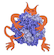IMP  2.4.0 The Integrative Modeling Platform
vector_generators.h File Reference

Functions to generate vectors. More...

#include "VectorD.h"
#include "Cylinder3D.h"
#include "Cone3D.h"
#include "Sphere3D.h"
#include "SpherePatch3D.h"
#include "BoundingBoxD.h"
#include "utility.h"
#include "internal/grid_range_d.h"
#include "internal/internal_vector_generators.h"Include dependency graph for vector_generators.h:This graph shows which files directly or indirectly include this file:

Go to the source code of this file.

## Namespaces

IMP
Synonym for IMP::kernel.

IMP::algebra
General purpose algebraic and geometric methods that are expected to be used by a wide variety of IMP modules.

## Functions

Vector3Ds IMP::algebra::get_uniform_surface_cover (const Sphere3Ds &in, double points_per_square_angstrom)

## Vector Generators

These functions generate vector objects. Some of the methods, those with random in their name, generate a single vector chosen uniformly from the specified domain. Others, the cover methods, generate a set of points distributed (somewhat) evenly over the domain.

template<int D>
VectorD< D > IMP::algebra::get_random_vector_in (const BoundingBoxD< D > &bb)
Generate a random vector in a box with uniform density. More...

template<int D>
VectorD< D > IMP::algebra::get_random_vector_on (const BoundingBoxD< D > &bb)
Generate a random vector on a box with uniform density. More...

template<int D>
VectorD< D > IMP::algebra::get_random_vector_in (const SphereD< D > &s)
Generate a random vector in a sphere with uniform density. More...

VectorD< 2 > IMP::algebra::get_random_vector_in (const SphereD< 2 > &s)

Vector3D IMP::algebra::get_random_vector_in (const Cylinder3D &c)
Generate a random vector in a cylinder with uniform density. More...

VectorD< 3 > IMP::algebra::get_random_vector_on_unit_sphere ()

template<int D>
VectorD< D > IMP::algebra::get_random_vector_on (const SphereD< D > &s)
Generate a random vector on a sphere with uniform density. More...

template<int D>
base::Vector< VectorD< D > > IMP::algebra::get_uniform_surface_cover (const SphereD< D > &s, unsigned int n)
Generate a set of vectors which covers a sphere uniformly. More...

Vector3Ds IMP::algebra::get_uniform_surface_cover (const Cylinder3D &cyl, int number_of_points)
Generate a set of 3d points that uniformly cover a cylinder. More...

template<int D>
base::Vector< VectorD< D > > IMP::algebra::get_uniform_upper_hemisphere_cover (const SphereD< D > &s, unsigned int n)
Generate a set of 3D points that uniformly cover a hemisphere. More...

Vector3Ds IMP::algebra::get_grid_surface_cover (const Cylinder3D &cyl, int number_of_cycles, int number_of_points_on_cycle)
Generate a grid of 3d points on a cylinder surface. More...

Vector3Ds IMP::algebra::get_uniform_surface_cover (const SpherePatch3D &sph, unsigned int number_of_points)
Generate a set of 3d points that uniformly cover a patch of a sphere. More...

Vector3Ds IMP::algebra::get_uniform_surface_cover (const Cone3D &cone, unsigned int number_of_points)

template<int D>
base::Vector< VectorD< D > > IMP::algebra::get_grid_interior_cover_by_spacing (const BoundingBoxD< D > &bb, double s)

Vector3Ds IMP::algebra::get_random_chain (unsigned int n, double r, const Vector3D &start=Vector3D(0, 0, 0), const Sphere3Ds &obstacles=Sphere3Ds())
Generate a random chain with no collisions. More...

## Detailed Description

Functions to generate vectors.